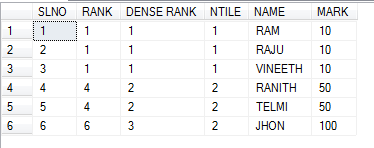### Ranking Functions In SQL Server

ROW_NUMBER ()
Row number returns continues number of row  with in a partition, starting at 1 for 1st Row
RANK ()
Ranking the each row with in the partition ,chance for gap depending partition
DENSE_RANK ()
Ranking the each row with in the partition without any gaps, means  continues ranking
NTILE()
Ranking the total records in a specific number of groups

CREATE TABLE TEST (NAME VARCHAR(20),MARK INT)

INSERT INTO TEST VALUES('RAM',10)
INSERT INTO TEST VALUES('RAJU',10)
INSERT INTO TEST VALUES('VINEETH',10)
INSERT INTO TEST VALUES('RANITH',50)
INSERT INTO TEST VALUES('TELMI',50)
INSERT INTO TEST VALUES('JHON',100)

SELECT
ROW_NUMBER() OVER (ORDER BY MARK) AS 'SLNO',
RANK() OVER (ORDER BY MARK) AS 'RANK',
DENSE_RANK() OVER (ORDER BY MARK) AS 'DENSE RANK',
NTILE(2) OVER (ORDER BY MARK) AS 'NTILE'
,NAME,MARK
FROM TEST

OUT PUT1.2.